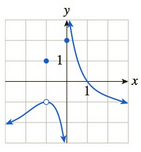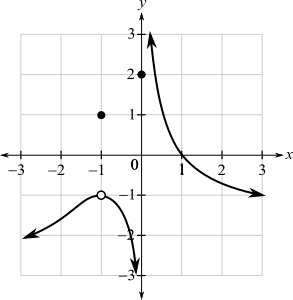Chapter 10.1, Problem 47EFinite Mathematics and Applied Cal...

7th Edition
Stefan Waner + 1 other
ISBN: 9781337274203

Solutions

Chapter
SectionFinite Mathematics and Applied Cal...

7th Edition
Stefan Waner + 1 other
ISBN: 9781337274203
Textbook Problem

In Exercises 35–48 the graph of f is given. Use the graph to compute the quantities asked for. [HINT: See Examples 4–5.]a. lim x → − 1 f ( x ) b. lim x → 0 + f ( x ) c. lim x → 0 − f ( x ) d. lim x → 0 f ( x ) e. f ( 0 ) f. f ( − 1 )(a)

To determine

The value of limx1f(x) by the use of the graph which is as follows:Explanation

Given information:

The graph is as follows:

From the graph, to evaluate the limit for x tends to 1 for the function f(x).

For this, take a point on the graph to the left of x=1 and move right along the curve, so that the x-coordinate approaches 1. Then, it is noticed from the graph that the function f(x) approaches the open dot at the height of 1.

Similarly, take a point on the graph to the right of x=1 and when move along the curve to the left so that the x-coordinate approaches 1

(b)

To determine

The value of limx0+f(x) by the use of the graph which is as follows:(c)

To determine

The value of limx0f(x) by the use of the graph which is as follows:(d)

To determine

The value of limx0f(x) by the use of the graph which is as follows:(e)

To determine

The value of f(0) by the use of the graph which is as follows:(f)

To determine

The value of f(1) by the use of the graph which is as follows:Still sussing out bartleby?

Check out a sample textbook solution.

See a sample solution

The Solution to Your Study Problems

Bartleby provides explanations to thousands of textbook problems written by our experts, many with advanced degrees!

Get Started

Prove Equation 4.

Single Variable Calculus: Early Transcendentals, Volume I

Show that if an 0 and limn nan 0, then an is divergent.

Single Variable Calculus: Early Transcendentals

True or False: f(x) = tan x is differentiable at x=2.

Study Guide for Stewart's Single Variable Calculus: Early Transcendentals, 8th